# CBSE Previous Year Question Papers Class 10 Maths Chapter Wise 2018 19 20

Here we are providing CBSE Previous Year Question Papers Class 6 to 12 solved with soutions CBSE Previous Year Question Papers Class 10 Maths Chapter wise 2018 19 20 class 10 Maths sample paper 2020 solved, sample paper class 10 Maths, cbse class 10 Maths question paper 2018, sample paper class 10 Maths 2019, Maths question paper for class 10, cbse previous year question papers class 10 Maths, cbse sample paper f Practice of previous year question papers and sample papers protects each and every student to score bad marks in exams.If any student of CBSE Board continuously practices last year question paper student will easily score high marks in tests. Fortunately earlier year question papers can assist the understudies with scoring great in the tests. Unraveling previous year question paper class 10 Maths is significant for understudies who will show up for Class 10 Board tests.

## Class 10 Subject Maths Paper Set 3 with Solutions

SECTION A

Question numbers 1 to 10 are multiple choice questions of 1 mark each. Select the correct choice.

Question 1: (Marks 1)

What is the largest number that divides 245 and 1029, leaving remainder 5 in each?

(a) 15

(b) 16

(c) 9

(d) 5

Answer :

(b) 16

Question 2: (Marks 1)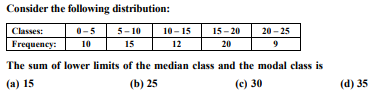Answer :

(b) 25

Question 3: (Marks 1)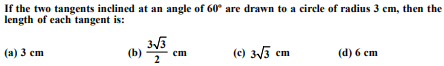Answer :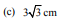Question 4: (Marks 1)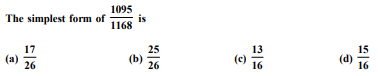Answer :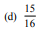Question 5: (Marks 1)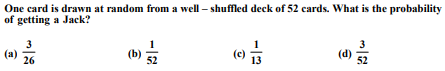Answer :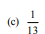Question 6: (Marks 1)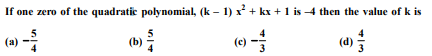Answer :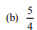Question 7: (Marks 1)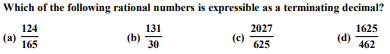Answer :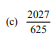Question 8: (Marks 1)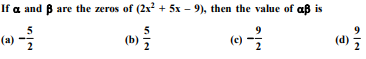Answer :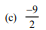Question 9: (Marks 1)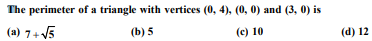Answer :

(d) 12

Question 10: (Marks 1)

If P(–1, 1) is the midpoint of the line segment joining A(–3, b) and B(1, b + 4), then b is equal to

(a) 1

(b) –1

(c) 2

(d) 0

Answer :

(b) –1

In Question numbers 11 to 15, fill in the blanks:

Question 11: (Marks 1)

Distance between (a, –b) and (a, b) is ________.

Answer :

2b units

Question 12: (Marks 1)

The value of k for which system of equations x + 2y = 3 and 5x + ky = 7 has no solution is ________.

Answer :

k = 10

Question 13: (Marks 1)

The value of (cos2 45º + cot2 45º) is ________.

Answer :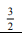Question 14: (Marks 1)

The value of (tan 27º – cot 63º) is ________.

Answer :

0

Question 15: (Marks 1)

If ratio of the corresponding sides of two similar triangles is 2:3, then ratio of their perimeters is _________.

Answer :

2 : 3

Answer the following questions, Question numbers 16 to 20

Question 16: (Marks 1)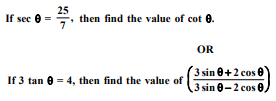Answer :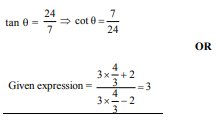Question 17: (Marks 1)

The perimeter of a sector of a circle of radius 14 cm is 68 cm. Find the area of the sector.

OR

The circumference of a circle is 39.6 cm. Find its area.

Answer :

l = 68 – 28 = 40 cm

A = 280 cm2

OR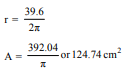Question 18: (Marks 1)

A letter of English alphabet is chosen at random. Determine the probability that chosen letter is a consonant.

Answer :

No. of consonents = 21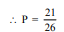Question 19: (Marks 1)

In Fig. 1, D and E are points on sides AB and AC respectively of a ΔABC such that DE || BC. If AD = 3.6 cm, AB = 10 cm and AE =4.5 cm, find EC and AC.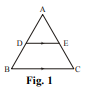Answer :

. EC = 8 cm

AC = 12.5 cm

Question 20: (marks 1)

If 3y – 1, 3y + 5 and 5y + 1 are three consecutive terms of an A.P., then find the value of y

Answer :

2(3y + 5) = 3y – 1 + 5y + 1

y = 5

SECTION B

Question numbers 21 to 26 carry 2 marks each.

Question 21: (Marks 2)

A bag contains 5 red, 8 white and 7 black balls. A ball is drawn at random from the bag. Find the probability that the drawn ball is

(i) red or white

(ii) not a white ball

Answer :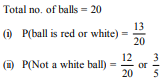Question 22: (Marks 2)

Two dice are thrown at the same time. Find the probability of getting different numbers on the two dice.

OR

Two dice are thrown at the same time. Find the probability that the sum of the two numbers appearing on the top of the dice is more than 9.

Answer :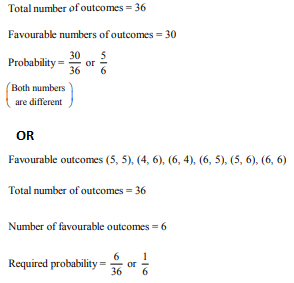Question 23: (Marks 2)

In Fig. 2, a circle is inscribed in a ΔABC, touching BC, CA and AB at P, Q and R respectively. If AB = 10 cm, AQ = 7 cm and CQ = 5 cm then find the length of BC.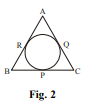Answer :

AQ = AR = 7 cm

BR = AB – AR = 10 – 7 = 3 cm

BC = BP + PC = BR + CQ

= 3 + 5 = 8 cm

Question 24: (Marks 2)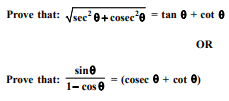Answer :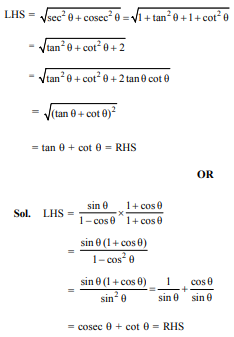Question 25: (Marks 2)

Three cubes each of volume 216 cm3 are joined end to end to form a cuboid. Find the total surface area of resulting cuboid.

Answer :

a3 = 216 cm3

a = 6 cm

TSA of cuboid = 5a2 + 4a2 + 5a2

= 14a2

= 504 cm2

Question 26: (Marks 2)

Find the values of p for which the quadratic equation x2 – 2px + 1 = 0 has no real roots.

Answer :

For no real roots

D < 0 (–2p)2 – 4 × 1 × 1 < 0

p2 – 1 < 0

–1 < p < 1

SECTION C

Question numbers 27 to 34 carry 3 marks each.

Question 27: (Marks 3)

If 1 and –2 are the zeroes of the polynomial (x3 – 4x2 – 7x + 10), find its third zero.

Answer :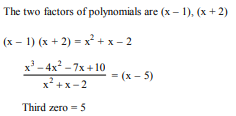Question 28: (Marks 3)

Draw a circle of radius 3 cm. From a point 7 cm away from its centre, construct a pair of tangents to the circle.

OR

Draw a line segment of 8 cm and divide it in the ratio 3 : 4.

Answer :

Drawing a circle of radius 3 cm, marking

Centre 0 and taking a point P such that

OP = 7 cm

Constructing two tangents

OR

Drawing a line segment of 8 cm

Dividing it in the ratio 3 : 4

Question 29: (Marks 3)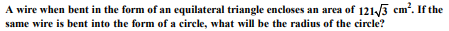Answer :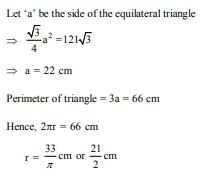Question 30: (Marks 3)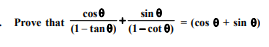OR

Prove that (sin θ + cosec θ)2 + (cos θ + sec θ)2 = 7 + tan2 θ + cot2 θ.

Answer :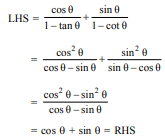OR

(sin θ + cosec θ)2 + (cos θ + sec θ)2

= sin2 θ + cosec2 θ + 2 + cos2 θ + sec2 θ + 2

= sin2 θ + 1 + cot2 θ + 2 + cos2 θ + 1 + tan2 θ + 2

= 7 + tan2 θ + cot2 θ

Question 31: (Marks 3)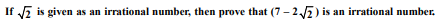OR

Find HCF of 44, 96 and 404 by prime factorization method. Hence find their LCM.

Answer :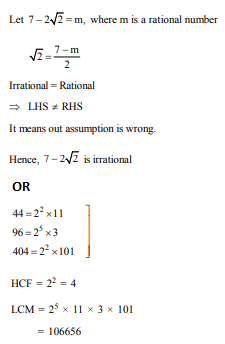Question 32: (Marks 3)

Prove that the parallelogram circumscribing a circle is a rhombus

Answer :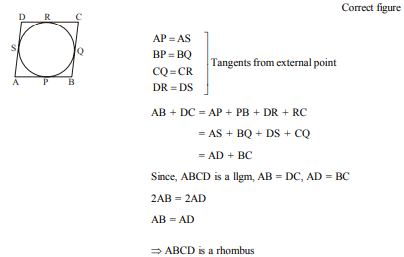Question 33: (Marks 3)

In Fig. 3, arrangement of desks in a classroom is shown. Ashima, Bharti and Asha are seated at A, B and C respectively. Answer the following:

(i) Find whether the girls are sitting in a line.

(ii) If A, B and C are collinear, find the ratio in which point B divides the line segment joining A and C.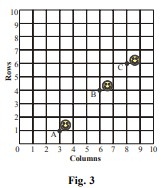Answer :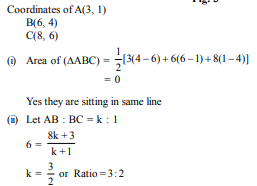Question 34: (Marks 3)

A number consists of two digits whose sum is 10. If 18 is subtracted from the number, its digit are reversed. Find the number.

Answer :

Let two digit number = 10x + y

x + y = 10 ...(i)

10x + y – 18 = 10y + x

⇒ x – y = 2 ...(ii)

On solving (i) & (ii) x = 6, y = 4

∴ Required number = 64

SECTION D

Question Nos. 35 to 40 carry 4 marks each.

Question 35: (Marks 4)

Some students planned a picnic. The total budget for food was ` 2,000 but 5 students failed to attend the picnic and thus the cost for food for each member increased by ` 20. How many students attended the picnic and how much did each student pay for the food?

Answer :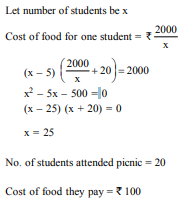Question 36: (Marks 4)

The sum of first 6 terms of an A.P. is 42. The ratio of its 10th term to 30th term is 1:3. Find the first and the 13th term of the A.P.

OR

Find the sum of all odd numbers between 100 and 300

Answer :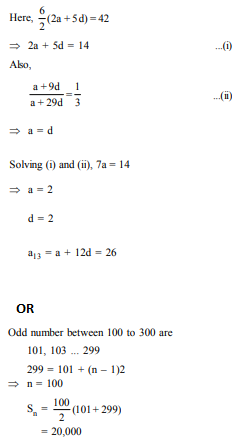Question 37: (Marks 4)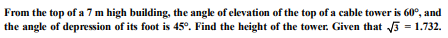Answer :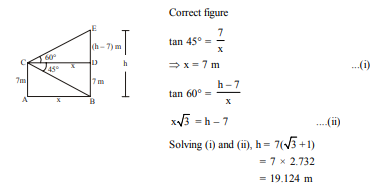Question 38: (Marks 4)

In a right triangle, prove that the square of the hypotenuse is equal to sum of squares of the other two sides.

OR

Prove that the tangents drawn from an external point to a circle are equal in length.

Answer :

For correct given, to prove, construction and figure

For correct proof

OR

For correct given, to prove, construction and figure

For correct proof

Question 39: (Marks 4)

A hemispherical depression is cut out from one face of a cubical wooden block of edge 21 cm, such that the diameter of the hemisphere is equal to edge of the cube. Determine the volume of the remaining block.

OR

A solid metallic cylinder of diameter 12 cm and height 15 cm is melted and recast into 12 toys in the shape of a right circular cone mounted on a hemisphere of same radius. Find the radius of the hemisphere and total height of the toy, if the height of the cone is 3 times the radius.

Answer :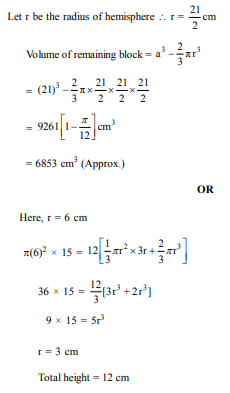Question 40: (marks 4)

Find the mean of the following data: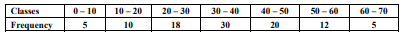Answer :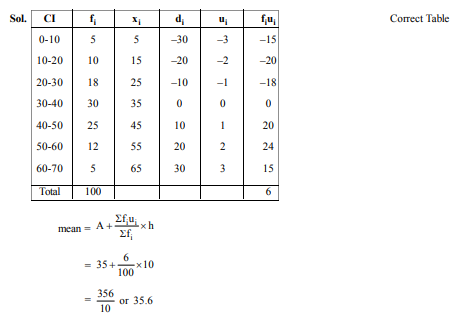☞ Click here for privious year Question papers

## class 10 Maths sample paper 2020 solved, sample paper class 10 Maths, cbse class 10 Maths question paper 2018, sample paper class 10 Maths 2019, Maths question paper for class 10, cbse previous year question papers class 10 Maths, cbse sample paper for class 10 Maths, cbse class 10 Maths question paper 2019, class 10 Maths sample paper 2019 solved, cbse class 10th Maths question paper, 10th Maths question paper, sample paper class 10 Maths 2020, Maths sample paper class 12, cbse question paper for class 9 Maths, cbse Maths sample paper, cbse 10th class Maths

### NCERT Books Free Pdf Download for Class 5, 6, 7, 8, 9, 10 , 11, 12 Hindi and English Medium

 Mathematics Biology Psychology Chemistry English Economics Sociology Hindi Business Studies Geography Science Political Science Statistics Physics Accountancy

## Please Share this webpage on facebook, whatsapp, linkdin and twitter.

Copyright @ ncerthelp.com A free educational website for CBSE, ICSE and UP board.